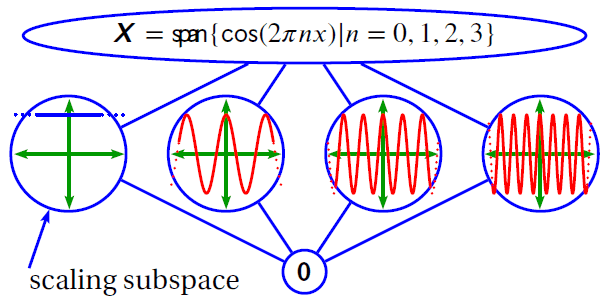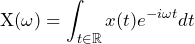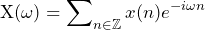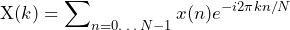# Fourier Analysis (FFT)

June 2, 2021

The fast Fourier transform (FFT) transforms time-domain data into the frequency-domain. It takes apart a single data waveform into many sine and cosine waves. This operation helps to reveal oscillations hidden in the original time data.

The FFT is a computational algorithm that efficiently implements a mathematical operation called the discrete-time Fourier transform (DTFT).

Like the FFT, the DTFT transforms time-domain data into the frequency domain. More specifically, the DTFT projects a length N time data sequence onto sinusoids at N different frequencies. The FFT performs the same mathematical operation as the DTFT in a computationally efficient manner.

#### Example

For example, for a length N=1024 data sequence, a DTFT operation would require about N2=1,048,576 operations, whereas the FFT would require about Nlog2N = 10,240 operations (about one-tenth as many).

However, this efficiency has a constraint—the FFT requires the length N to be a power of 2. Due to such, all analysis line values are a power of 2.

### FFT & Fourier Analysis

The FFT is one of several Fourier analysis methods. In turn, Fourier analysis is one of many analysis methods that take apart data x(t) collected in the time domain. These components are typically projections of the data onto a set of basis functions.For Fourier analysis, the operation that takes apart data using projections is the Fourier transform operator. The resulting set of components is the Fourier transform of x(t).

A Fourier transform basis function is any function ϕ(ω)=e-iωt, where ω can be any real number (any element in ℝ).

By Euler’s identity, each basis function ϕ(ω) has a real and an imaginary sinusoidal part: ϕ(ω) = e-iωt = cos(ωt) + i sin(ωt).

### Fourier Operators

There are several Fourier operators, including:

• Fourier Transform (FT): maps from continuous to continuous

(1)• Discrete Time Fourier Transform (DTFT): maps from discrete to continuous

(2)• Discrete Fourier Transform (DFT): maps from discrete to discrete

(3)